# High School Math : How to find the slope of a perpendicular line

## Example Questions

### Example Question #1 : How To Find The Slope Of A Perpendicular Line

A line passes through the points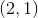and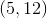.  If a new line is drawn perpendicular to the original line, what will its slope be?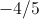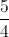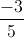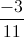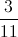Explanation:

The original line has a slope of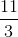, a line perpendicular to the original line will have a slope which is the negative reciprocal of this value.

### Example Question #2 : Perpendicular Lines

Which of the following is the equation of a line that is perpendicular to the line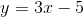?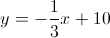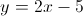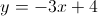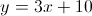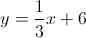Explanation:

Perpendicular lines have slopes that are the opposite reciprocals of each other. Thus, we first identify the slope of the given line, which is(since it is in the form, whererepresents slope).

Then, we know that any line which is perpendicular to this will have a slope of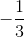.

Thus, we can determine that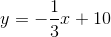is the only choice with the correct slope.

### Example Question #3 : Perpendicular Lines

What will be the slope of the line perpendicular to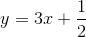?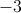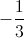Explanation:

In standardform, theis the slope.

The slope of a perpendicular line is the negative reciprocal of the original line.

For our given line, the slope is. Therefore, the slope of the perpendicular line is.

### Example Question #1 : How To Find The Slope Of A Perpendicular Line

Which of the following is perpendicular to the line described by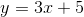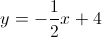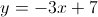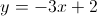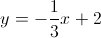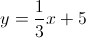Explanation:

The definition of perpendicular lines is that their slopes are inverse reciprocals of one another. Since the slope in the given equation is, this means that the slope of its perpendicular line would be.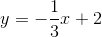is the only equation listed that has a slope of.

### Example Question #2 : How To Find The Slope Of A Perpendicular Line

Which of the following is perpendicular to the line described by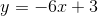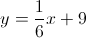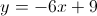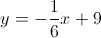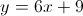Explanation:

The definition of perpendicular lines is that their slopes are inverse reciprocals of one another. Since the slope in the given equation is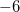, this means that the slope of its perpendicular line would be.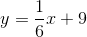is the only equation listed that has a slope of.

### Example Question #3 : How To Find The Slope Of A Perpendicular Line

Which of the following gives the slope of a line that is perpendicular to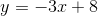?Explanation:

Recall that the slopes of perpendicular lines are opposite reciprocals of one another. As a result, we are looking for the opposite reciprocal of. Thus, we can get that the opposite reciprocal is### Example Question #4 : How To Find The Slope Of A Perpendicular Line

Find the slope of the line perpendicular to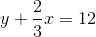.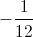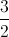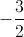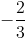Explanation:

Put this equation into slope-intercept form, y = mx + b, to find the slope, m.

Do this by subtracting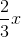from both sides of the equation: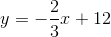The slope of this line is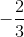.

The slope of the perpendicular line is the negative reciprocal. Switch the numerator and denominator, and then multiply by: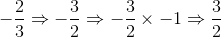### Example Question #5 : How To Find The Slope Of A Perpendicular Line

What is the slope of the line perpendicular to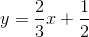?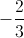Explanation:

In standardform,is the slope.

The slope of a perpendicular line is the negative reciprocal of the original line.

For our given line, the slope is. Therefore, the slope of the perpendicular line is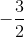.

### Example Question #41 : Coordinate Geometry

Find the slope of this line: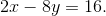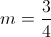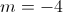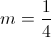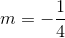Isolate forso that the equation now reads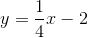The slope is